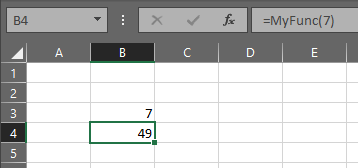top of page

# When to use a Sub vs a Function in VBA?

There are 2 ways to write VBA code. You can write your code in either a Sub or a so calledFunction. A Sub is often also referred to as a procedure and is something that simply executes your code. A Function is however something that runs your code, but returns a variable at the end of the execution. it also often combined with an input parameter as the purposes is to convert this input parameter to something.

### 1. Sub

```           Sub MyScript ()
'Do some code.
End Sub```

### ​2. Function

```           Function MyScript ('input variables to the functions)
'Do some code.
End Function```

### Explanation of the difference between Subs and Functions

The way I look at it, is that Subs are places to store and execute your code, whereas Functions are dependent on an Input variable and you are creating an output variable which is the name of your function. In the above example MyScript is the output variable and it is not defined, which means it is a variant. If you want to define it it could look like the below where the name is MyFunc and it is of variable type double and we are multiplying each input number by 7.

```            Function MyFunc (x As Double) As Double
MyFunc = x * 7
End Function```

Functions are great for low code end user input as they are accessible from the worksheet, alternatively they are pretty nifty if you call them from your sub instead of writing repetitive kinda similar code. By calling a Sub or Function from your current Sub, your main script looks a lot cleaner and significantly less rows. This means that it is also much easier and quicker to amend something should that be needed in the future.

In the below picture we can see that we are simply typing "=MyFunc(7)" to access the function where we input 7, and our result is 49.Now if we try to mimic the Function with a Sub it could look something like this. This multiplies our current cell value by 7 and we are triggering the our Sub or Script via the "WriteToCell" button.

```Sub WriteToCell()

'ActiveCell.Value is our current value 7.
ActiveCell.Value = ActiveCell.Value * 7
​
End Sub```